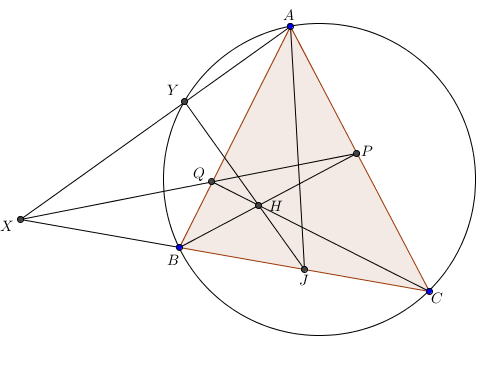# Find The Length

Geometry Level 5Let $\triangle ABC$ be a triangle with $AB= 6, BC = 7, CA=8.$ Let $P,Q$ be the feet of perpendiculars from $B,C$ on $CA,AB$ respectively. Let $BP$ and $CQ$ meet at $H,$ and let $PQ$ and $BC$ meet at $X.$ Line $AX$ meets the circumcircle of $\triangle ABC$ at $Y,$ where $Y \neq A.$ Lines $HY$ and $BC$ intersect at $J.$ Given that $AJ^2 = \dfrac{m}{n}$ for some coprime positive integers $m,n,$ find $m+n.$

×